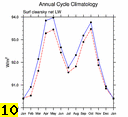f.e15a6.FAMIPC5.ne30_ne30.Club_Test.012
and
f.e15a6.FAMIPC5.ne30_ne30.Club_Test.014

DIAG Set 10 - Annual cycle line plots of global means

Surface Variables
PBLH Planetary boundary layer height plot
PSL Sea level pressure plot
TREFHT 2-meter air temperature plot
TS Surface temperature plot

Constant Pressure Levels
CHI 200 200 mb velocity potential plot
EKE 850 850 mb transient eddy kinetic energy plot
PSI 200 200 mb stream function plot
T 850 850 mb temperature plot
T 200 200 mb temperature plot
U 200 200 mb zonal wind plot
VT 850 850 mb meridional heat transport plot
Z 500 500 mb geopotential height plot
Z 300 300 mb geopotential height plot

Hydrologic Cycle
EP Evaporation - precipitation plot
ICEFRAC Sea-ice area (Northern) plot
ICEFRAC Sea-ice area (Southern) plot
PRECST Snowfall rate (Northern) plot
PRECST Snowfall rate (Southern) plot
PRECT Precipitation rate plot
PREH2O Total precipitable water plot
QFLX Surface water flux plot
SNOWHICE Snow depth on sea-ice (Northern) plot
SNOWHICE Snow depth on sea-ice (Southern) plot
SNOWHLND Snow depth on land (Northern) plot
SNOWHLND Snow depth on land (Southern) plot

Surface Energy Fluxes
FLDS Downwelling longwave flux plot
FLNS Net longwave flux plot
FLNSC Clearsky net longwave flux plot
FSDS Downwelling shortwave flux plot
FSNS Net shortwave flux plot
FSNSC Clearsky net shortwave flux plot
LHFLX Latent heat flux plot
RESSURF Residual energy flux plot
SHFLX Sensible heat flux plot

Top of Model Energy Fluxes
FLNT Net longwave flux plot
FLNTC Clearsky net longwave flux plot
FSNT Net shortwave flux plot
FSNTC Clearsky net shortwave flux plot
LWCF Longwave cloud forcing plot
SOLIN Incoming solar radiation plot
SWCF Shortwave cloud forcing plot

Cloud Variables
CLDHGH High-level cloud amount plot
CLDLOW Low-level cloud amount plot
CLDMED Mid-level cloud amount plot
CLDTOT Total cloud amount plot
TGCLDIWP Total grid-box cloud liquid water path (ice) plot
TGCLDLWP Total grid-box cloud liquid water path (liquid) plot

CFMIP Observation Simulator Package (COSP) Primary Diagnostics

ISCCP-COSP From D1 Daytime Jul1983-Sep2008

CALIPSO GOCCP 2007-2010
CLDTOT_CAL Total cloud amount plot
CLDLOW_CAL Low-level cloud amount plot
CLDMED_CAL Mid-level cloud amount plot
CLDHGH_CAL High-level cloud amount plot

ISCCP-COSP From D1 Daytime Jul1983-Sep2008
CLDTOT_ISCCPCOSP Total cloud amount (tau > 0.3) plot
CLDTHICK_ISCCPCOSP Optically thick cloud amount (tau > 23.0) plot

MISR L3 CTH-OD V5 Mar2000-Nov2009
CLDTOT_MISR Total cloud amount (tau > 0.3) plot
CLDTHICK_MISR Optically thick cloud amount (tau > 23.0) plot

MODIS-COSP 2003-2010
CLDTOT_MODIS Total cloud amount (tau > 0.3) plot
CLDTHICK_MODIS Optically thick cloud amount (tau > 23.0) plot

CALIPSO GOCCP 2007-2010
CLDTOT_CAL Total cloud amount plot
CLDLOW_CAL Low-level cloud amount plot
CLDMED_CAL Mid-level cloud amount plot
CLDHGH_CAL High-level cloud amount plot

CLOUDSAT-COSP From Level 2 GEOPROF Jun2006-Nov2010
CLDTOT_CS2 Total cloud amount plot

ISCCP-COSP From D1 Daytime Jul1983-Sep2008
CLDTOT_ISCCPCOSP Total cloud amount (tau > 0.3) plot
CLDLOW_ISCCPCOSP Low-topped cloud amount (tau > 0.3) plot
CLDMED_ISCCPCOSP Mid-topped cloud amount (tau > 0.3) plot
CLDHGH_ISCCPCOSP High-topped cloud amount (tau > 0.3) plot
CLDTHICK_ISCCPCOSP Optically thick cloud amount (tau > 23.0) plot
MEANPTOP_ISCCPCOSP Mean cloud top pressure plot
MEANCLDALB_ISCCPCOSP Mean cloud albedo plot

MISR L3 CTH-OD V5 Mar2000-Nov2009
CLDTOT_MISR Total cloud amount (tau > 0.3) plot
CLDLOW_MISR Low-topped cloud amount (tau > 0.3) plot
CLDMED_MISR Mid-topped cloud amount (tau > 0.3) plot
CLDHGH_MISR High-topped cloud amount (tau > 0.3) plot
CLDTHICK_MISR Optically thick cloud amount (tau > 23.0) plot

MODIS-COSP 2003-2010
CLDTOT_MODIS Total cloud amount (tau > 0.3) plot
CLDLOW_MODIS Low-topped cloud amount (tau > 0.3) plot
CLDMED_MODIS Mid-topped cloud amount (tau > 0.3) plot
CLDHGH_MODIS High-topped cloud amount (tau > 0.3) plot
CLDTHICK_MODIS Optically thick cloud amount (tau > 23.0) plot
CLIMODIS Total ice cloud amount plot
CLWMODIS Total liquid cloud amount plot
IWPMODIS Mean ice water path plot
LWPMODIS Mean liquid water path plot
PCTMODIS Mean cloud top pressure plot
REFFCLIMODIS Mean effective radius (ice clouds) plot
REFFCLWMODIS Mean effective radius (liquid clouds) plot
TAUILOGMODIS Log-weighted mean optical thickness (ice clouds) plot
TAUWLOGMODIS Log-weighted mean optical thickness (liquid clouds) plot
TAUTLOGMODIS Log-weighted mean optical thickness (all clouds) plot
TAUIMODIS Mean optical thickness (ice clouds) plot
TAUWMODIS Mean optical thickness (liquid clouds) plot
TAUTMODIS Mean optical thickness (all clouds) plot